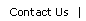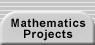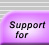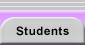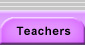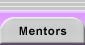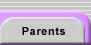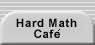# The Chinese Remainder Theorem

The Chinese Remainder Theorem states that for relatively prime m1, m2, ... , there is a unique solution (mod m1m2...) to the system of congruences

x = a1 (mod m1)
x = a2 (mod m2)
...

For a discussion and some sample problems, see Chinese Remainder Theorem.Translations of mathematical formulas for web display were created by tex4ht. © Copyright 2003 Education Development Center, Inc. (EDC)